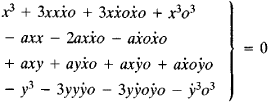# Fluxions, Method of

The following article is from The Great Soviet Encyclopedia (1979). It might be outdated or ideologically biased.

## Fluxions, Method of

the earliest form of differential and integral calculus. It was originally developed by I. Newton, who conceived its basic principles in 1665 and 1666.

Newton formulated the basic problems of the method in terms of the space traversed by a local motion: “(1) Given the length of the space continuously (that is, at every instant of time), to find the speed of motion at any time proposed. (2) Given the speed of motion continuously, to find the length of the space described at any time proposed” (I. Newton, Matematicheskie raboty, Moscow-Leningrad, 1937, p. 45). He regarded time as the independent variable, on which other variables depend. He applied the term “fluents” to the quantities x, y, z, . . . that vary simultaneously and continuously over time. The rates of change of fluents were called by him fluxions: ẋ, ẏ, ż,. . .. In other words, fluxions are the derivatives of fluents with respect to time. Newton referred to infinitely small changes in fluents as moments. The moment thus corresponds to what we now call the differential. The moment of the independent variable was denoted by the symbol o, and that of the fluent x, by xo.

An idea of the basic procedure for finding fluxions and of the peculiarities of Newton’s notation can be obtained from the following example (ibid., p. 50): “Let any equation

x3axx + axyy3 = 0

be given, and substitute x + ẋo in place of x and y + ẏo in place of y. There will ariseNow by hypothesis x3axx + axyy3 = 0; when these terms are erased and the remaining terms are divided by o, there will remain

3xxẋ – 2axẋ + ayẋ + axẏ – 3yyẏ + 3xẋẋoaẋẋo + aẋẏo – 3yẏẏo + 3oo3oo = 0

But whereas o is supposed to be infinitely small so that it may express the moments of quantities, the terms that are multiplied by it will be equivalent to nothing in respect of the others. I therefore cast them out, and there remains

3xxẋ – 2axẋ + ayẋ + axẏ – 3yyẏ = 0.”

The inverse problem (that is, the determination of fluents), the foundations of the method of fluxions, and the history of the method are described in NEWTON, ISAAC and DIFFERENTIAL CALCULUS.

The method of fluxions with its distinctive notation constituted a special form of differential and integral calculus and was used only by British mathematicians. In the late 17th and early 18th centuries it was supplanted by differential calculus, whose notation was more convenient and was therefore more often used. The symbols used in the method of fluxions have been partially retained in mechanics and vector analysis.

### REFERENCES

Newton, I. Matematicheskie raboty. Moscow-Leningrad, 1937. (Translated from Latin.)
Newton, I. Matematicheskie nachala natural’noi filosofii. Moscow-Leningrad, 1936. (Translated from Latin.)
Zeuthen, H. G. Istoriia matematiki v XVI i XVII vekakh, 2nd ed. Moscow-Leningrad, 1938. (Translated from German.)
Kolmogorov, A. N. “N’iuton i sovremennoe matematicheskoe myshlenie.” In Moskovskii universitet—pamiati Isaaka N’iutona, 1643–1943. Moscow, 1946.
Cajori, F. A History of the Conceptions of Limits and Fluxions in Great Britain, from Newton to Woodhouse. Chicago-London, 1919.